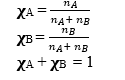# Mole Fraction Formula, Definition and Examples

## Mole Fraction Definition :-

Mole fraction is defined as the ratio of moles of one components to the total no. of moles of all the components present in solution.
It is a unit less and dimension less quantity.

## Mole Fraction formula :-

If a substance A of nA moles is dissolves in solution of B having nB ,then mole fraction is expressed as denoted by greek letter $\chi$ (chi)
For two componentswhere, n = moles
$moles = \frac {Weight }{Molar Mass}$

## Example Questions based on Mole Fraction Formula

Question 1
Find the mole fraction of Methanol CH3OH and water in a solution prepared by dissolving 4.5 g of alcohol in 40 g of H2O.
Molar Mass of H2O is 18gm/mole and Molar mass of CH3OH is 32gm/mole
Solution
Moles of CH3OH = 4.5 / 32 = 0.14 mole
Moles of H2O = 40 / 18 = 2.2 moles
Therefore, according to the equation
Mole fraction of CH3OH = 0.14 / 2.2 + 0.14
= 0.061
Mole fraction of H2O. = 2.2 / 2.2 + 0.14
= 0.940

Question 2
Calculate the mole fraction of HCl and H2O in a solution of hydrochloric acid in water, containing 30% HCl by weight.
Solution
The solution contains 30 grams of Hydrochloric acid and 70 grams of water. Also,
Molar mass of HCl is 36.5 grams/mole.
Molar mass of water is 18 grams/mole.
Moles of HCl = 30/36.5 = .821 moles of HCl
Moles of water = 70/18 = 3.88 moles of H2O.
Mole fraction of HCl = .821/(.821 + 3.88) = .174
Mole fraction of H2O = 3.88/(.821 + 3.88) = .825
Question 3
In a solution , we have 1 mole ofA , 1 mole ofB and 2 mole of C. Find the mole fraction of each of them
Solution
This is an extension of two component solution which we discussed above
Mole fraction will be defined as
χA = nA / (nA + nB + nC ) = 1/(1+1+2) =.25
χB = nB / (nA + nB + nC ) = 1/(1+1+2) =.25
χC = nC / (nA + nB + nC ) = 2/(1+1+2) =.5
Also
χA + χB + χC =1

## Quiz based on mole fraction and its formula

### Quiz Time

Question 1 Calculate the mole fraction of solvent in a solution where mole fraction of solute is .3.
A) 1
B) .7
C) .2
D) 1.3
Question 2 In a solution we have 2 mol of Substance A, 2 mol of Substance B, and 7 mol of Substance C.Find the the mole fraction of Substance C?
A) .63
B) .1
C) 1
D) None of the above
Question 3 what is the unit of mole fraction
A) moles
B) unit-less
C) gm/moles
D) None of the above
Question 4 What is the mole fraction of substance A in solution if 30 grams of Substance A is dissolved in 3.25 moles of Substance B?
Given Molar Mass of substance A =30 g/moles
A) .21
B) .12
C) .541
D) .235
Question 5 What is the mole fraction of Substance B in a mixture that is 50.0% weight of substance A in Substance B (Molar Mass of A = 60.06 g/mol; Molar mass of B = 148.16 g/mol)?
A) .2885
B) .1234
C) .12
D) .34
Question 6The sum of the mole fraction of all the components in a solution is
A) 0
B) 1
C) -1
D) None of the above